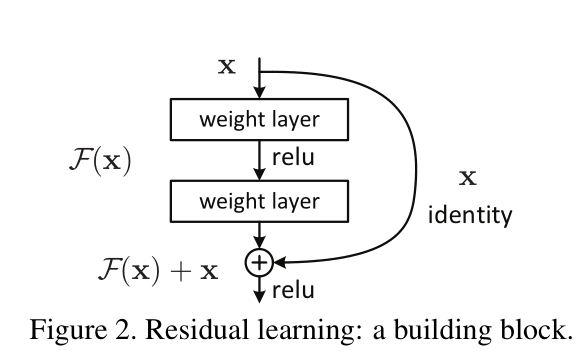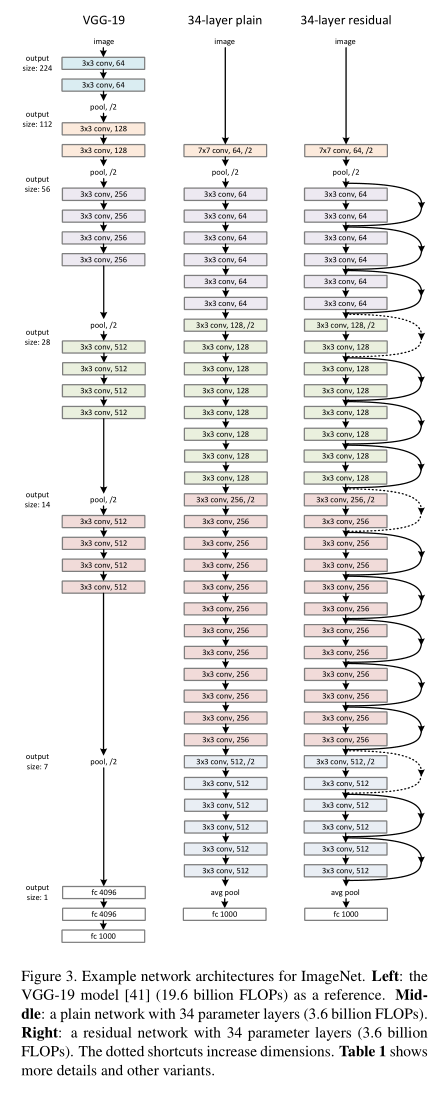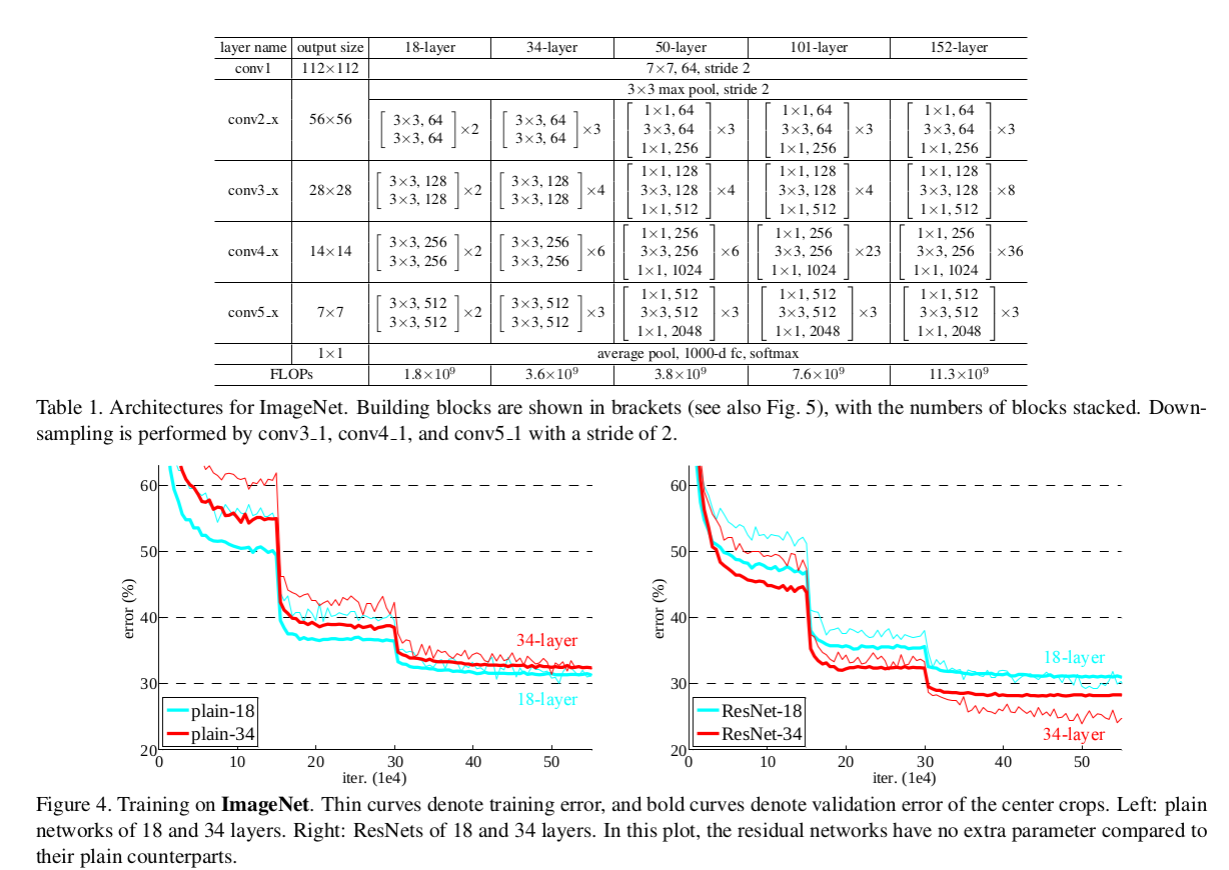## Summary

In this blog spot I'm presenting a few performance measures for machine learning tasks. These performance measures come up a lot in the marketing domain.

Take at home lessons:
• the measure you optimize to makes a difference
• the measure you report makes a difference
• use measure appropriate for problem/community
• accuracy often is not sufficient/appropriate
• only accuracy generalizes to >2 classes
• this is not an exhaustive list of performance measures

## Confusion Matrix

First we construct a confusion matrix for a binary classification problem. Given a classification function f(x)->R and a threshold T that can split the outcomes into {0, 1} we can create a confusion matrix that counts the occurrences of the predicted class given the true label.

## Accuracy

Accuracy is then measured as the percentage of correct responses (True Positives + True Negatives) over the total amount of responses.

### Problems with Accuracy

This measure is commonly used but it can be misleading. The problems arise from the domain we are modelling. If one of the class for example is poorly represented into the metric is meaningless as we could predict the same class always and still have a good results.

• Assumes equal cost for both kinds of errors
• cost(b-type-error) = cost (c-type-error)
• is 99% accuracy good?
• can be excellent, good, mediocre, poor, terrible
• depends on problem
• Base Rate = accuracy of predicting predominant class

### Weighted (Cost sensitive) Accuracy

A modified version of accuracy is "Weighted Accuracy" were we count the cost of misclassification.

In this scenario we aiming for a model and a threshold that can minimize the total cost.

### Lift

If we are not interested in the accuracy on the entire dataset but want accurate predictions for 5%, 10% or 20% of the dataset then we can use the lift measure.
Lift measures how much better than random prediction on the fraction of the dataset predicted true (f(x) > threshold).

### Precision / Recall

• The Precision measure counts how many of the interest class are correct.
• The Recall measure counts how many of the interest class does the model return.
In the case below the interest class is a(1).

We can change the sweep over the threshold calculate Precision/Recall multiple times and graph out what is called the Precision/Recall curve.

At each different threshold we can see a different tradeoff between the two metrics.
• When the threshold is too high then c (everything is predicted as class 0) becomes zero and then the precision becomes zero.
• When the threshold is too low then b (everything is predicted as class 1) becomes zero and then the recall becomes zero.
Both of these metrics are flawed in isolation and it is the eye of the modeller on which one better represents the problem.

### F-Measure

The F-Measure is an attempt to merge the two measures to construct a more meaningful performance measure.

• Developed in WWII to statistically model false positive and false negative detections of radar operators
• Better statistical foundations than most other measures
• Standard measure in medicine and biology
• Becoming more popular in ML

Although ROC graphs are apparently simple, there are some common misconceptions and pitfalls when using them in practice.

One of the earliest adopters of ROC graphs in machine learning was Spackman (1989), who demonstrated the value of ROC curves in evaluating and comparing algorithms.

ROC graphs are conceptually simple, but there are some non-obvious complexities that arise when they are used in research.

#### ROC Plot

• Sweep threshold and plot
• TPR vs. FPR
• Sensitivity vs. 1-Specificity
• P(true|true) vs. P(true|false)
• Sensitivity = a/(a+b) = Recall = LIFT numerator
• 1 - Specificity = 1 - d/(c+d)

A ROC graph depicts relative trade-offs between benefits (true positives) and costs (false positives).

• The lower left point (0,0) represents the strategy of never issuing a positive classiffication.
• The opposite strategy is represented by the upper right point (1,1).
• The point (0,1) represents perfect classiffication.
• The diagonal line y = x represents the strategy of randomly guessing a class.
• A random classifier will produce an ROC point that "slides" back and forth on the diagonal based on the frequency with which it guesses the positive class. In order to get away from this diagonal into the upper triangular region, the classifier must exploit some information in the data.
• Any classifier that appears in the lower right triangle performs worse than random guessing. This triangle is therefore usually empty in ROC graphs.
• ROC curves have an attractive property: they are insensitive to changes in class distribution.
• Any performance metric that uses values from both columns of theconfusion matrix will be inherently sensitive to class skews. Metrics such as accuracy, precision, lift and F scores use values from both
columns of the confusion matrix.
• ROC graphs are based upon TP rate and FP rate, in which each dimension is a strict columnar ratio, so do not depend on class distributions.

## Thursday, October 24, 2019

### Model Distillation

Model Distillation is the process of taking a big model or ensemble of models and producing a smaller model that captures most of the performance of the original bigger model. It could be also better be described as a blind model replication method.

The reasons for doing so are:
1. improved run-time performance (FLOP operations)
2. (maybe) better generalization because of the model simplicity
3. you don't have access to the training of the original model.
4. you have access to a remotely deployed model and you want to replicate it (it happens more than you can imagine)
5. original model maybe is too complicated
6. insights that may arise from the process itself

### How it works

Assume a MNIST classifier $$F_{MNIST}$$ composed of an ensemble of $$N$$ convolutional deep neural networks that produces a logit $$z_i$$ which is then converted to a probability of an input image, $$x_i$$, for each of the possible labels $$C_{0}-C_{9}$$.

The distillation process will give us an  $$F_{MNIST_{distilled}}$$ composed of a single deep neural network that will approximate the classification results of the bigger ensemble of models.

In distillation, knowledge is transferred from the teacher model to the student by minimizing a loss function in which the target is the distribution of class probabilities predicted by the teacher model. That is - the output of a softmax function on the teacher model's logits.

Logits $$z_j$$ are converted to probabilities $$P(C_i|x)$$ using the softmax layer:

$$p_i = \frac {exp(z_i)} {\sum_{j}exp(z_j)}$$

However, in many cases, this probability distribution has the correct class at a very high probability, with all other class probabilities very close to 0. As such, it doesn't provide much information beyond the ground truth labels already provided in the dataset.

To tackle this issue, Hinton et al., 2015 introduced the concept of "softmax temperature". The probability $$q_i$$ is computer by the logit $$z_i$$ for the scalar softmax temperature $$T$$:

$$q_i = \frac {exp(\frac{z_i}{T})} {\sum_{j}exp(\frac{z_j}{T})}$$

where T is a temperature that is normally set to 1. Using a higher value for T produces a softer probability distribution over classes. Softer probability distribution means that the values are somewhat diffused and a 0.999 probability may become 0.9 and the rest spread to the other classes.

In the simplest form of distillation, knowledge is transferred to the distilled model by training it on a transfer set and using a soft target distribution for each case in the transfer set that is produced by using the cumbersome model with a high temperature in its softmax. The same high temperature is
used when training the distilled model, but after it has been trained it uses a temperature of 1. When the correct labels are known for all or some of the transfer set, this method can be significantly improved by also training the distilled model to produce the correct labels. One way to do this is to use the correct labels to modify the soft targets, but we found that a better way is to simply use a weighted average of two different objective functions.
1. The first objective function is the cross entropy with the soft targets and this cross entropy is computed using the same high temperature in the softmax of the distilled model as was used for generating the soft targets from the cumbersome model.
2. The second objective function is the cross entropy with the correct labels. This is computed using exactly the same logits in softmax of the distilled model but at a temperature of 1.
This very simple operation can have a multitude of knobs and parameters to adjust but the core essence is very simple and works quite well.

## Friday, October 18, 2019

### ResNets inner workings and notes

A residual network (or ResNet) is a standard deep neural net architecture, with state-of-the-art performance across numerous applications. The main premise of ResNets is that they allow the training of each layer to focus on fitting just the residual of the previous layer’s output and the target output. Thus, we should expect that the trained network is no worse than what we can obtain if we remove the residual layers and train a shallower network instead.

Up until 2015 we had 3 mainstream ways of training deep networks:
• Greedy per layer optimization and freezing
• Various flavors of Dropout
• and Batch Normalization (came out early 2015, wasn't mainstream until 2016, now it is patented by google so who knows if people can use it)
The main contribution of the "Deep Residual Learning for Image Recognition, 2015" paper is a novel  and smart building block for training very deep neural networks.

### The "Residual Learning" or "Identity Learning" block.A special note here:
We need at least 2 weight layers here (nonlinearities are not essential but welcome) to get the benefits of the universal function approximator that has been proven since the 90's. You can add more but you then hit the limits of information propagation and you will need residual/skip connections inside the residual block.

A stack of $$n$$ residual blocks is described as follows :

$$x_0$$ : input
$$x_1 = x_0 + F_1(x_0)$$
$$x_2 = x_1 + F_2(x_1)$$
$$x_3 = x_2 + F_3(x_2)$$
$$...$$
$$x_n = x_{n-1} + F_{n-1}(x_{n-1})$$

This can be re-written as  :

$$x_n = x_{n-1} + F_{n-1}( x_{n-2} + F_{n-2}(x_{n-2})$$

Which can be expanded as :

$$x_n = x_{n-1} + F_{n-1}( x_{n-2} + F_{n-2}( x_{n-3} +F_{n-3}( x_{n-4} + F_{n-4}(... + F_1(x_0))))))$$

Now if we assume that $$F_i$$ is a linear function (it is not for $$x \lt 0$$ ) but we can ignore it) :

$$x_n = x_{n-1} + F_{n-1}( x_{n-2} ) + F_{n-1}F_{n-2}(x_{n-3}) + F_{n-1}F_{n-2}F_{n-3}(x_{n-4}) + ... F_{n-1}F_{n-2}F_{n-3}...F_1(x_0)$$

As we can see from the equations and the equivalent graph, there is a clear information flow from the raw data to the output. This means that the major pain point of vanishing gradient is avoided.This design when stacked as above allows the gradient to flow through the whole network bypassing any tricky points and in essence training the deeper network properly. Information bottlenecks can be introduced by design but the gradient can flow from the loss layer up to the base layer.

Residual Networks (ResNets) have been used to train up to a 1000 layers. It was proven empirically that going deeper generalizes better than shallow wide networks. Again empirically several variations of the Residual Block are tried:In practice the Residual Function is not 2 (convs or fully connected) weight layers but they are accompanied by a Batch Normalization layer. This helps stabilize the gradient and the co-variate shift (there are various opposing hypothesis on why batch norm works).

In the paper "Identity Mappings in Deep Residual Networks, 2016" a different layout was proposed that improved the performance. We can see an inversion of the order of the layers. The architecture, the analysis and the system design remains the same though.
This new arrangements produces better results on very deep networks for the CIFAR dataset, shown below. Also the convergence is much faster. Since they are practically the same from an engineering perspective we don't have a reason to reject the one with the better performance.